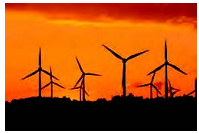Chapter 9.3, Problem 18ESMathematical Excursions (MindTap C...

4th Edition
Richard N. Aufmann + 3 others
ISBN: 9781305965584

Solutions

Chapter
SectionMathematical Excursions (MindTap C...

4th Edition
Richard N. Aufmann + 3 others
ISBN: 9781305965584
Textbook Problem

Wind Energy In a recent year, wind machines in the United States generated 181.7 billion kWh of electricity, enough to serve over 16 million households. The nation’s total electricity production that year was 4094 billion kWh. (Source: Energy Information Administration) What percent of the total energy production was generated by wind machines? Round to the nearest tenth of a percent.To determine

To calculate: The percent of electricity generated by wind machines in US, rounded to the nearest tenth of a percent when in a recent year, wind machines in the United States generated 181.7 billion kWh of electricity, enough to serve over 16 million households. The nation’s total electricity production that year was 4094 billion kWh. (Source: Energy Information Administration)

Explanation

Given Information:

United States generated 181.7 billion kWh of wind energy from the total of 4094 billion kWh.

Formula used:

Proportion used for percent:

percent100=amountbase

Calculation:

Base is 4094 and amount is 181.7 ,

The electricity generated by wind machines,

percent100=

Still sussing out bartleby?

Check out a sample textbook solution.

See a sample solution

The Solution to Your Study Problems

Bartleby provides explanations to thousands of textbook problems written by our experts, many with advanced degrees!

Get Started